NCERT Exemplar - MCQs

Chapter 5 Class 12 Continuity and Differentiability
Serial order wise

## (d) None of these

This question is similar to Ex 5.1, 32 - Chapter 5 Class 12 and Ex 5.2, 9 - Chapter 5 Class 12 - Continuity and Differentiability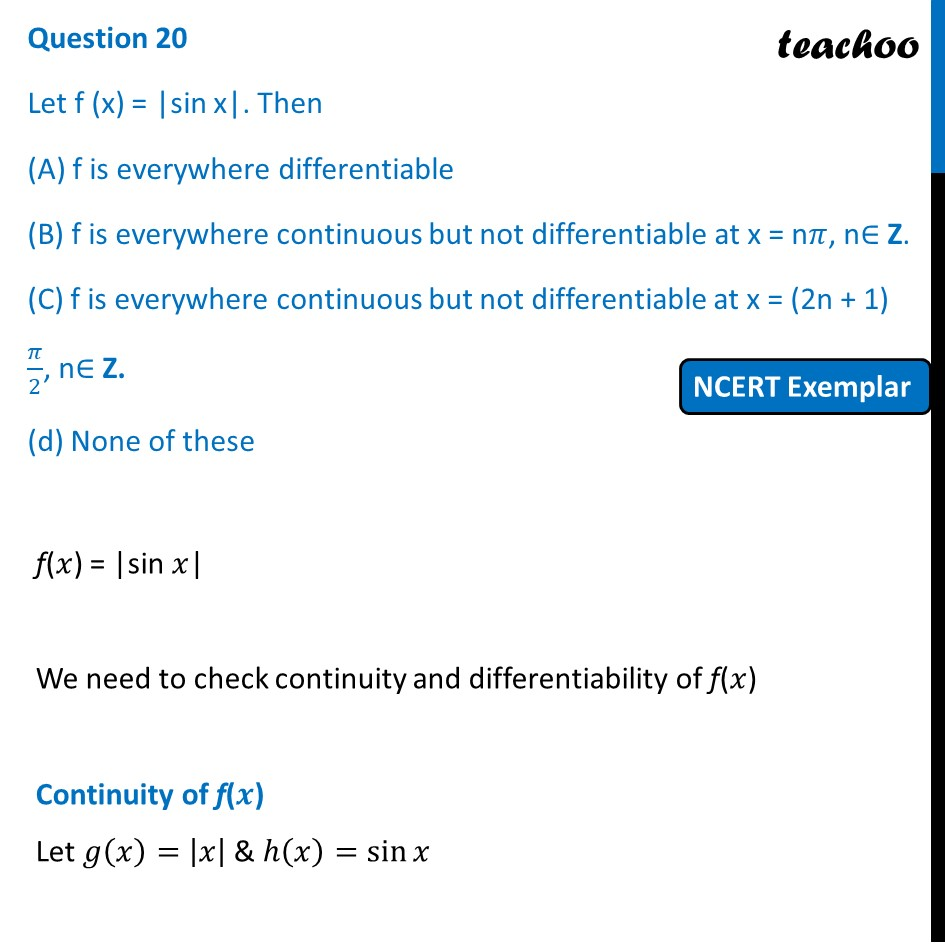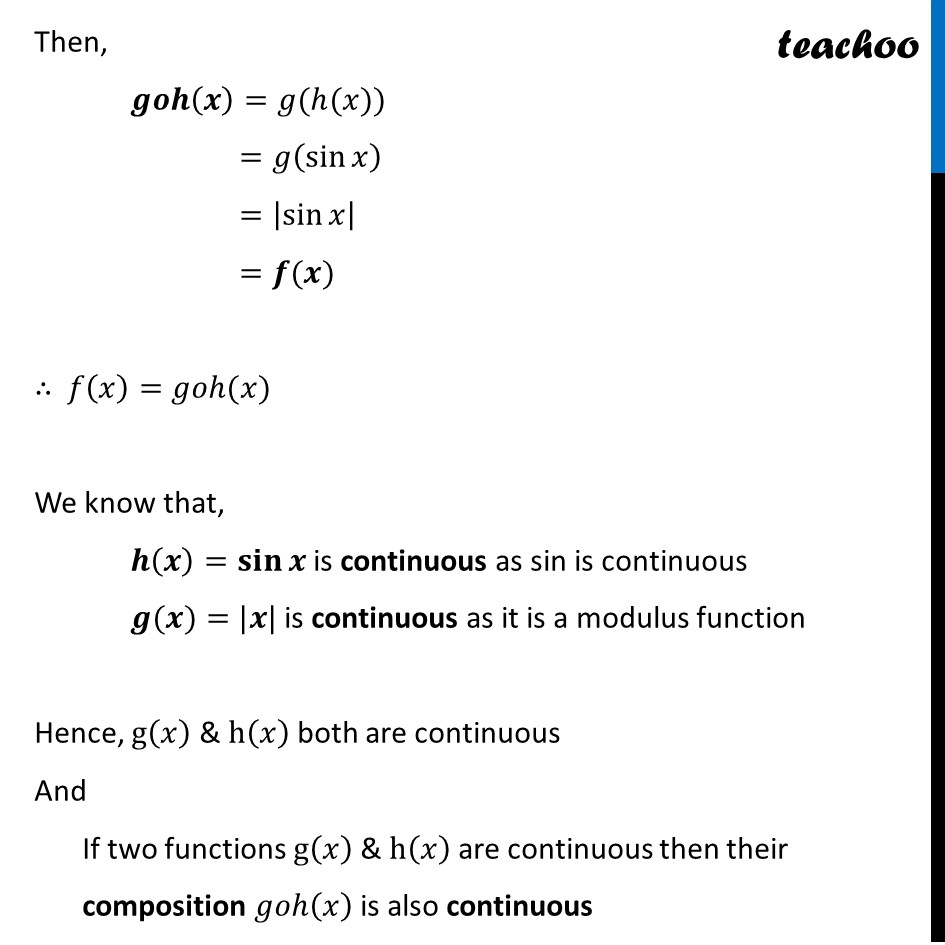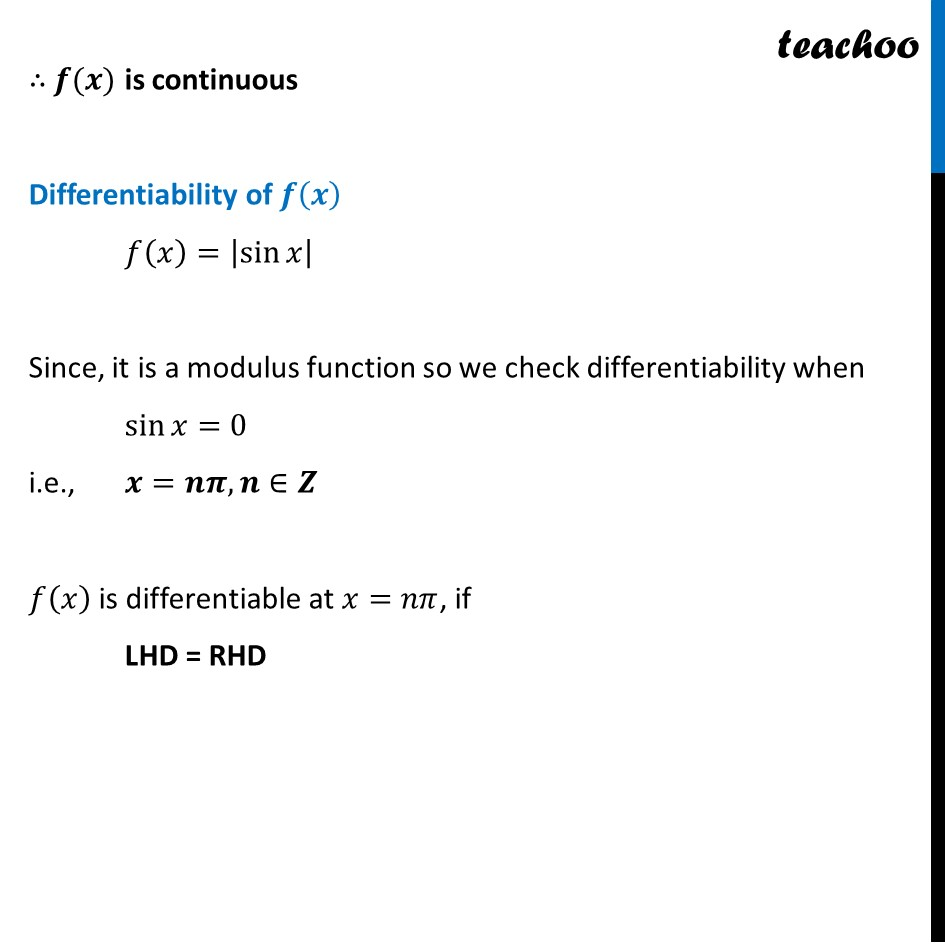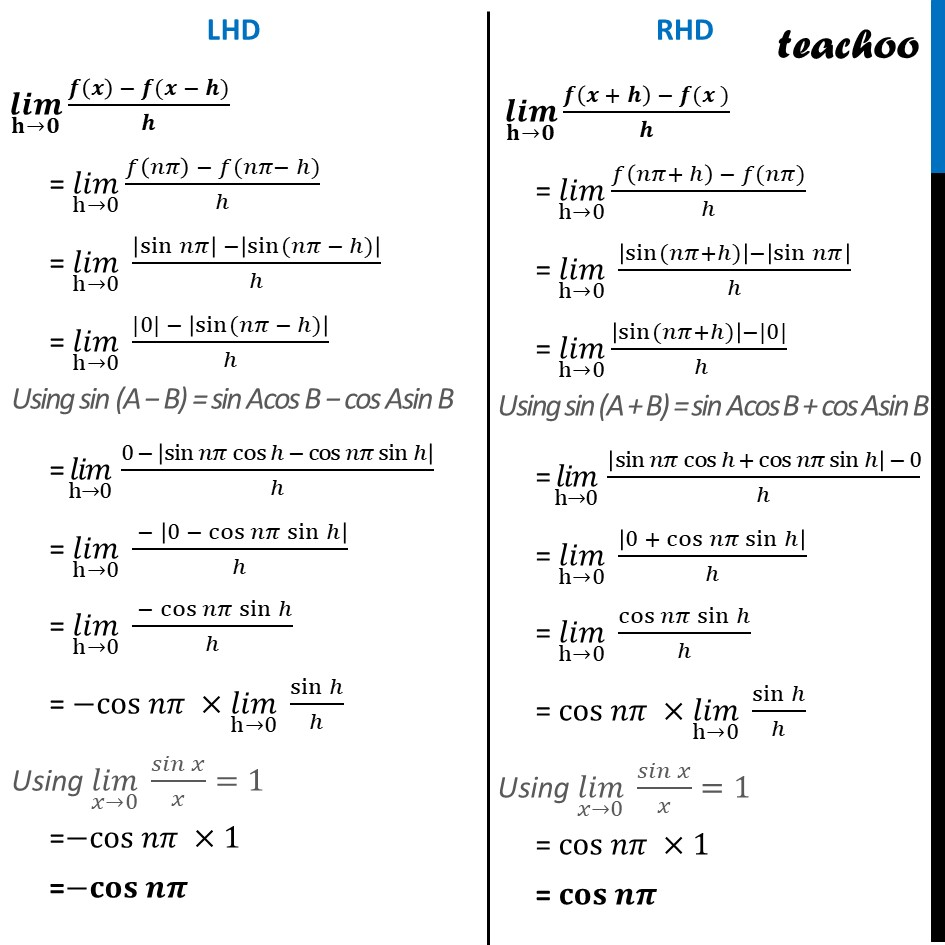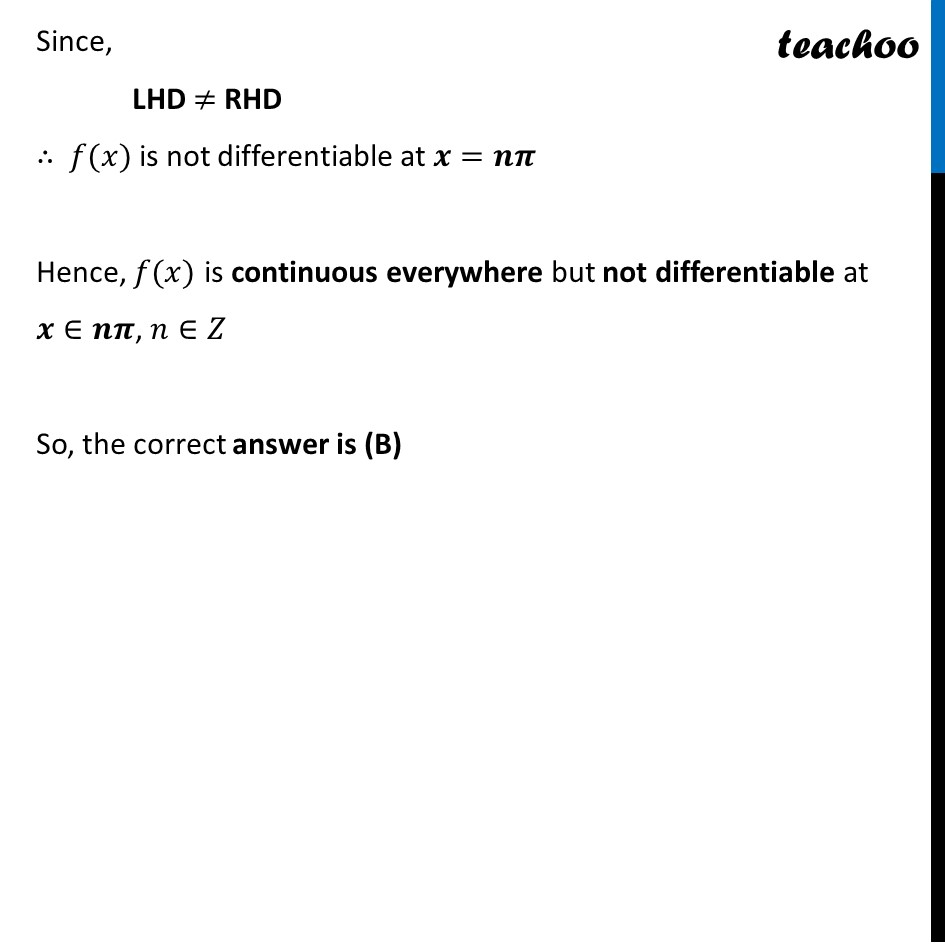Learn in your speed, with individual attention - Teachoo Maths 1-on-1 Class

### Transcript

Question 18 Let f (x) = |sin x|. Then (A) f is everywhere differentiable (B) f is everywhere continuous but not differentiable at x = n𝜋, n∈ Z. (C) f is everywhere continuous but not differentiable at x = (2n + 1) 𝜋/2, n∈ Z. (d) None of these f(𝑥) = |sin 𝑥| We need to check continuity and differentiability of f(𝑥) Continuity of f(𝒙) Let 𝑔(𝑥)=|𝑥| & ℎ(𝑥)=sin⁡𝑥 Then, 𝒈𝒐𝒉(𝒙)=𝑔(ℎ(𝑥)) =𝑔(sin⁡𝑥 ) =|sin⁡𝑥 | =𝒇(𝒙) ∴ 𝑓(𝑥)=𝑔𝑜ℎ(𝑥) We know that, 𝒉(𝒙)=𝐬𝐢𝐧⁡𝒙 is continuous as sin is continuous 𝒈(𝒙)=|𝒙| is continuous as it is a modulus function Hence, g(𝑥) & h(𝑥) both are continuous And If two functions g(𝑥) & h(𝑥) are continuous then their composition 𝑔𝑜ℎ(𝑥) is also continuous ∴ 𝒇(𝒙) is continuous Differentiability of 𝒇(𝒙) 𝑓(𝑥)=|sin⁡𝑥 | Since, it is a modulus function so we check differentiability when sin⁡𝑥=0 i.e., 𝒙=𝒏𝝅, 𝒏∈𝒁 𝑓(𝑥) is differentiable at 𝑥=𝑛𝜋, if LHD = RHD (𝒍𝒊𝒎)┬(𝐡→𝟎) (𝒇(𝒙) − 𝒇(𝒙 − 𝒉))/𝒉 = (𝑙𝑖𝑚)┬(h→0) (𝑓(𝑛𝜋) − 𝑓(𝑛𝜋− ℎ))/ℎ = (𝑙𝑖𝑚)┬(h→0) (|sin⁡𝑛𝜋 | −|sin⁡〖(𝑛𝜋 − ℎ)〗 |)/ℎ = (𝑙𝑖𝑚)┬(h→0) (|0| − |sin⁡〖(𝑛𝜋 − ℎ)〗 |)/ℎ Using sin (A − B) = sin Acos B − cos Asin B = (𝑙𝑖𝑚)┬(h→0) (0 − |sin⁡〖𝑛𝜋 cos⁡〖ℎ − cos⁡〖𝑛𝜋 sin⁡ℎ 〗 〗 〗 |)/ℎ = (𝑙𝑖𝑚)┬(h→0) ( − |0 − cos⁡〖𝑛𝜋 sin⁡ℎ 〗 |)/ℎ = (𝑙𝑖𝑚)┬(h→0) ( −cos⁡〖𝑛𝜋 sin⁡ℎ 〗)/ℎ = −cos 𝑛𝜋 ×(𝑙𝑖𝑚)┬(h→0) sin⁡ℎ/ℎ Using (𝑙𝑖𝑚)┬(𝑥→0) 𝑠𝑖𝑛⁡𝑥/𝑥=1 =−cos 𝑛𝜋 ×1 =−𝐜𝐨𝐬 𝒏𝝅 (𝒍𝒊𝒎)┬(𝐡→𝟎) (𝒇(𝒙 + 𝒉) − 𝒇(𝒙 ))/𝒉 = (𝑙𝑖𝑚)┬(h→0) (𝑓(𝑛𝜋+ ℎ) − 𝑓(𝑛𝜋))/ℎ = (𝑙𝑖𝑚)┬(h→0) (|sin⁡〖(𝑛𝜋+ℎ)〗 |−|sin⁡𝑛𝜋 |)/ℎ = (𝑙𝑖𝑚)┬(h→0) (|sin⁡〖(𝑛𝜋+ℎ)〗 |−|0|)/( ℎ) Using sin (A + B) = sin Acos B + cos Asin B = (𝑙𝑖𝑚)┬(h→0) (|sin⁡〖𝑛𝜋 cos⁡〖ℎ + cos⁡〖𝑛𝜋 sin⁡ℎ 〗 〗 〗 | − 0)/ℎ = (𝑙𝑖𝑚)┬(h→0) |0 + cos⁡〖𝑛𝜋 sin⁡ℎ 〗 |/ℎ = (𝑙𝑖𝑚)┬(h→0) cos⁡〖𝑛𝜋 sin⁡ℎ 〗/ℎ = cos 𝑛𝜋 ×(𝑙𝑖𝑚)┬(h→0) sin⁡ℎ/ℎ Using (𝑙𝑖𝑚)┬(𝑥→0) 𝑠𝑖𝑛⁡𝑥/𝑥=1 = cos 𝑛𝜋 ×1 = 𝐜𝐨𝐬 𝒏𝝅 Since, LHD ≠ RHD ∴ 𝑓(𝑥) is not differentiable at 𝒙=𝒏𝝅 Hence, 𝑓(𝑥) is continuous everywhere but not differentiable at 𝒙∈𝒏𝝅, 𝑛∈𝑍 So, the correct answer is (B)